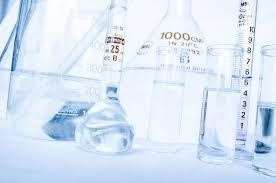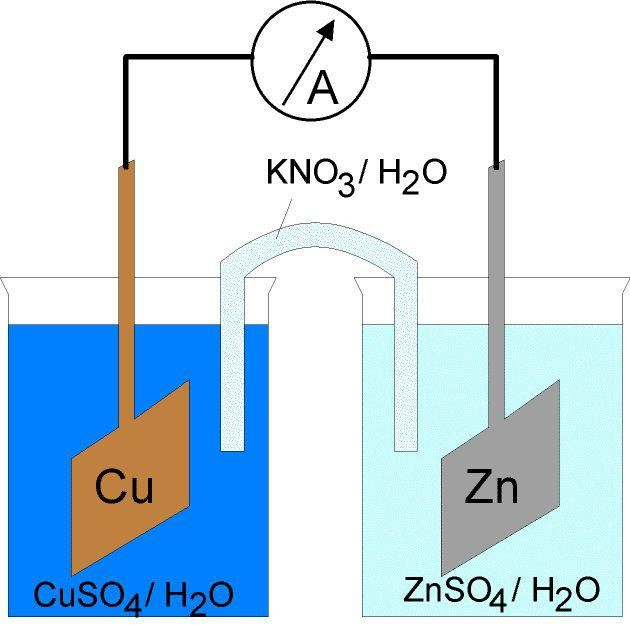Looking for revision notes that are specific to the exam board you are studying? If so, click the links below to view our condensed, easy-to-understand revision notes for each exam board, practice exam question booklets, mindmap visual aids, interactive quizzes, PowerPoint presentations and a library of past papers directly from the exam boards.

# Dilution

## Key Information & Overview:

• Definitions
• What is dilution?
• Why Dilution is important?
• Dilution in Electrolysis

### Definition

Dilution is the act of adding a solvent, usually water, to a solution.

### What is dilution?

Dilution is when you add a solvent to a solution. In A level Chemistry, dilution only occurs with water. The amount of water added to a solute will change its concentration. A solution that is concentrated has less water added to it. It usually has a concentration of more than 1 mole per dm3(mol/dm3). A solution that is dilute has more water added into it. A solution that is dilute usually has a concentration of less than 1 mole per dm3(mol/dm3).The amount of water added into a solute will only change the volume of the solute and thus the concentration of the solute will change. However, the moles of the solute and the mass of the solute will not change. The moles and the mass of the solute will remain the same.

For example, you put in 4 tablespoons of sugar in a glass of water (250 ml of water). The water becomes very sweet. We can say that the solution is concentrated. Then you add 3 more glasses of water into the solution. The water now becomes less sweet as the solution has diluted. The concentration of the sugar changed but it is to be noted that the tablespoons of sugar remained the same. The 4 tablespoons of sugar now moved about in a liter of water rather than a glass of water.### Why Dilution is important?

Dilution of solutions helps us in many ways. During titration, solutions are diluted so there is a larger volume of solution available. This allows the students to conduct multiple titrations without the fear of running out the solution. Solutions are also diluted because acids, at higher concentrations, are very corrosive to the skin and can have permanent effects. So, for safety reasons as well, solutions are diluted.

Question

25 cm3of KmnO4​​ at the concentration of 0.1 mol/dm3 is pipetted and added to the beaker. That 25 cm3 is diluted with water till the volume in the beaker is 100 cm3. Find the concentration of KMnO​​4 in the beaker.

Solution

The first statement indicates that KmnO4 ​had the original concentration of 0.1 mol/dm3. This can also be written as per 1 dm3(1000 cm3) of water, there are 0.1 moles of KMnO​4. By using the

ratio method, we can find out the number of moles in the 25 cm3sample that was pipetted out.

 Moles Volume (cm3​) 0.1 1000 x 25

By doing cross multiplication we can calculate the number of moles.

X = (0.1)(25)/1000

X = 0.0025 moles

0.0025 moles of KMnO​​were taken out when 25 cm3of the solution was pipetted out.

Then, the 25 cm3was made into 100 cm3by adding water. As we discussed earlier, adding water only makes the concentration and the volume change. The moles and the mass of the solute remain as is. So, the 100 cm3will have the same number of moles as the 25 cm3that was pipetted out. Now, we know that 100 cm3will have 0.0025 moles of KMnO4​​ in it, we can calculate its concentration using the ratio method.

 Moles Volume (cm3​) 0.0025 100 x 1000

As concentration is measured in moles/dm3and dm3equals to 1000 cm3, we can calculate the concentration by putting 1000 cm3in the volume column and finding the corresponding number

of moles. That will be the concentration of KMnO​​4 after dilution.

X = (0.0025)(1000)/100

X = 0.025 moles/dm3

The concentration of KmnO4 ​​in the beaker is 0.025 moles.

### Dilution in Electrolysis

The other concept of dilution comes in Electrolysis. As you will learn about Electrolysis in detail, in the coming chapters, we will just lightly go through the electrolysis and how dilution comes into play without going into detail.

As studied in O levels, the cations move to the cathode and the anions move to the anode. When the concentrations were different, the more concentrated one got discharged from the electrode. We will study the same thing in A levels but this time we will have values assigned to them called E . This value represents the tendency of an ion to go to the cathode. The higher the value, the more likely it will go to the cathode. E is measured in Volts.The E values of different ions are measured at standard conditions. One of the standard condition is that the ion is at a concentration of 1 mol/dm3.

Changing the concentration of the ion changes the E value of the ion and thus changes its tendency to go to the cathode. The change in the E value is calculated with an equation called the “Nerst’s equation”. The formula will be given when needed in the question paper.

E = E    + (0.059/z) log [oxidised species]/[reduced species]

Z = no. of electrons used

[ ] = concentration of the ion or atom inside

## Summary:

• Dilution is the addition of water to reduce concentration
• Dilution does not change the number of moles
• Dilution changes the E value of the atoms or ions during electrolysis